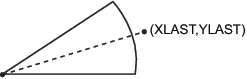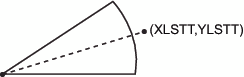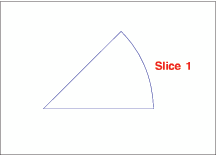# CNTL2TXT Function

Copies the values of the internal coordinates stored in the variable pairs (XLAST, YLAST) to (XLSTT, YLSTT).

## Syntax

FUNCTION='CNTL2TXT';

## Details

You can use CNTL2TXT to calculate the position of labels on a graph. For example, the following DATA step uses CNTL2TXT to position a pie slice label in the center of the arc and just beyond the arc itself, as shown in
First, use the PIE function to draw the pie slice:
```data pielabel;
retain xsys ysys "3";
length function style \$ 8;
function="pie"; size=20; x=30; y=30;
style="empty"; rotate=45; output;```
Then use the PIEXY function to calculate a point outside of the arc as shown in
```/* find a point that is half of the arc (rotate*.5) */
/* and is 4 units beyond the radius (size=1.1) */
function="piexy"; angle=rotate*.5; size=1.1; output;```
Position Calculated with the PIEXY FunctionAt this point, the XLAST and YLAST variables contain the coordinates of the point that is calculated by PIEXY. However, (XLAST, YLAST) cannot be used directly by text functions. Use CNTL2TXT to copy the coordinates in (XLAST, YLAST) to the XLSTT and YLSTT variables, which text functions can use. Coordinates After Using the CNTL2TXT Function shows the results.
```function="cntl2txt";
output;```
Coordinates After Using the CNTL2TXT FunctionNow you can use the LABEL function to write the label as shown in Specify missing values for the X and Y variables to force LABEL to use the XLSTT and YLSTT variables instead of the X and Y variables.
```/* write the label "Slice 1" and position it to     */
/* the right of the point stored in XLSTT and YLSTT */
function="label"; text="Slice 1"; angle=0; rotate=0;
position="6"; style="swissb"; size=4; x=.; y=.;
output;
run;
/* draw the Annotate graphics */
proc ganno anno=pielabel;
run;
quit;```
Labeled Pie Slice